## Example Questions

### Example Question #1 : X And Y Intercept

Find the slope of the following line: 6x – 4= 10

1.5

–1.5

5/2

–5/2

1.5

Explanation:

Putting the equation in y = mx + b form we obtain y = 1.5x – 2.5.

The slope is 1.5.

### Example Question #2 : X And Y Intercept

What is the x-intercept of the line in the standardcoordinate plane for the following equation?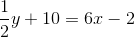3

212

-24

2

Explanation:

This question is asking us to find the x-intercept. Remember that the y-value is equal to zero at the x-intercept. Substitute zero in for the y-variable in the equation and solve for the x-variable.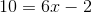Add 2 to both sides of the equation.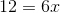Divide both sides of the equation by 6.The line crosses the x-axis at 2.

### Example Question #3 : X And Y Intercept

What is the equation of a line that has an x-intercept of 4 and a y-intercept of -6?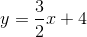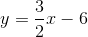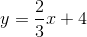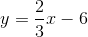Explanation:

The equation of a line can be written in the following form:In this formula, is the slope, and b represents the y-intercept. The problem provides the y-intercept; therefore, we know the following information: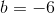We can calculate the slope of the line, because if any two points on the function are known, then the slope can be calculated. Generically, the slope of a line is defined as the function's rise over run, or more technically, the changes in the y-values over the changes in the x-values.  It is formally written as the following equation:The problem provides the two intercepts of the line, which can be written as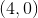and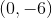. Substitute these points into the equation for slope and solve:.

Substitute the calculated values into the general equation of a line to get the correct answer:.